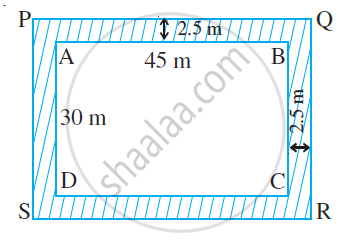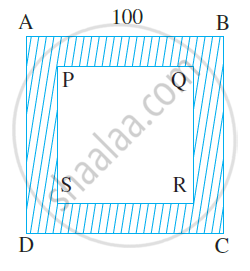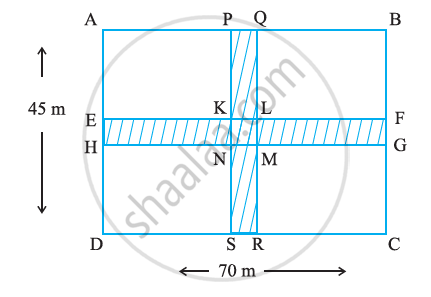# Problems based on Perimeter and Area

#### Example

A rectangular park is 45 m long and 30 m wide. A path 2.5 m wide is constructed outside the park. Find the area of the path.Let ABCD represent the rectangular park and the shaded region represents the path 2.5 m wide.

To find the area of the path, we need to find (Area of rectanglePQRS – Area of rectangle ABCD).

We have,
PQ = (45 + 2.5 + 2.5) m = 50 m
PS = (30 + 2.5 + 2.5) m = 35 m

Area of the rectangle ABCD = l × b = 45 × 30 m2 = 1350 m2

Area of the rectangle PQRS = l × b = 50 × 35 m2 = 1750 m2

Area of the path = Area of the rectangle PQRS − Area of the rectangle ABCD

= (1750 − 1350) m2 = 400 m2.

#### Example

A path 5 m wide runs along inside a square park of side 100 m. Find the area of the path. Also, find the cost of cementing it at the rate of Rs. 250 per 10 m2.Let ABCD be the square park of side 100 m. The shaded region represents the path 5 m wide.

PQ = 100 – (5 + 5) = 90 m

Area of square ABCD = (side)2 = (100)2 m2 = 10000 m2

Area of square PQRS = (side)2 = (90)2 m2 = 8100 m2.

Therefore, area of the path = (10000 − 8100) m2 = 1900 m2

Cost of cementing 10 m2 =Rs. 250

Therefore, cost of cementing 1 m2 = Rs. 250/10

So, cost of cementing 1900 m2 = Rs. 250/10 xx 1900 = Rs. 47,500.

#### Example

Two crossroads, each of width 5 m, run at right angles through the center of a rectangular park of length 70 m and breadth 45 m and parallel to its sides. Find the area of the roads. Also, find the cost of constructing the roads at a rate of Rs. 105 per m2.Area of the crossroads is the area of shaded portion, i.e., the area of the rectangle PQRS and the area of the rectangle EFGH. But while doing this, the area of the square KLMN is taken twice, which is to be subtracted.

Now,
PQ = 5 m and PS = 45 m
EH = 5 m and EF = 70 m
KL = 5 m and KN = 5 m

Area of the path = Area of the rectangle PQRS + area of the rectangle EFGH – Area of the square KLMN

= PS × PQ + EF × EH – KL × KN

= (45 × 5 + 70 × 5 − 5 × 5) m2

= (225 + 350 − 25) m2 = 550 m2

Cost of constructing the path = Rs. 105 × 550 = Rs. 57,750.

If you would like to contribute notes or other learning material, please submit them using the button below.